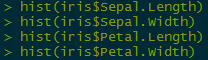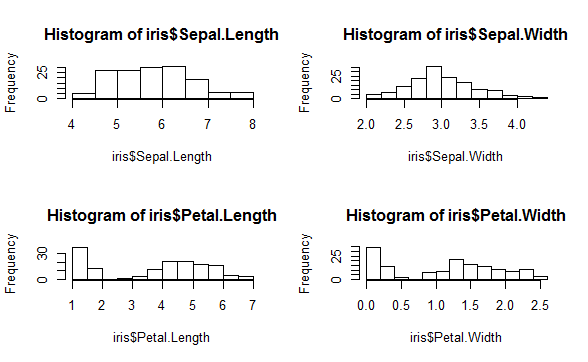# How would you make multiple plots onto a single page in R?

Plotting multiple plots onto a single page using base graphs is quite easy:

For, example if you want to plot 4 graphs onto the same pane, you can use the below command:

``````par(mfrow=c(2,2))
``````